Introduction to Tableau and Data Visualization
)}

# Scatter Plots --- Discount-Profit Relationship

We typically use scatter plots to show a relationship between two measures. In this section, we will try to find a relationship between profit and discount.

## 1. The Detail Mark

First, drag discount to columns, and profit to rows.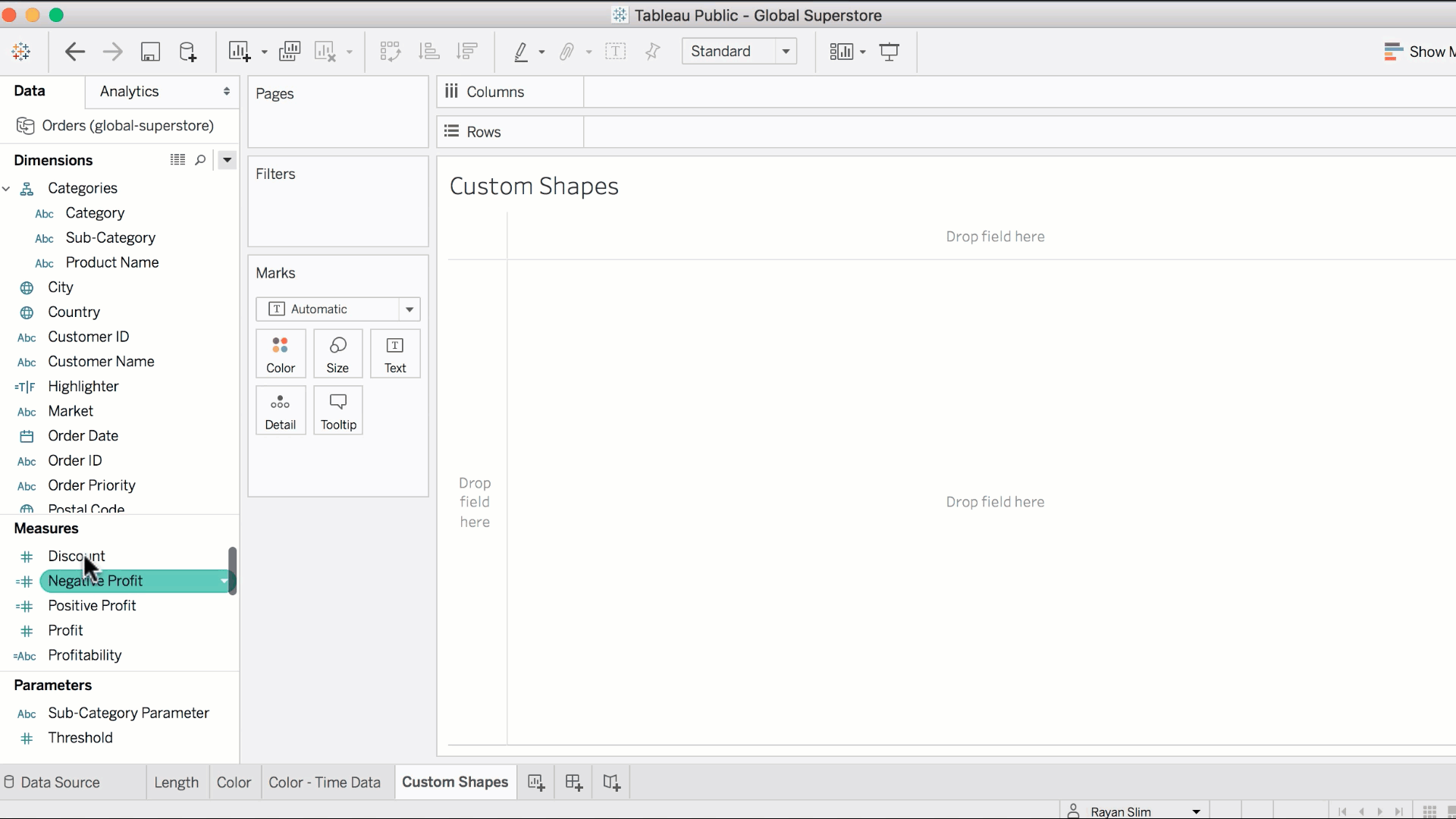Whenever you drag two measures to the row and column shelves, Tableau resorts to a scatter plot visualization.

Notice how we only see a single point. That is because Tableau is aggregating the sum of profits across all records; and the sum of discounts across all records.

First of all, it doesn't make any sense to take the sum of discounts. If you were to view the data, you'd notice that discounts are percentages.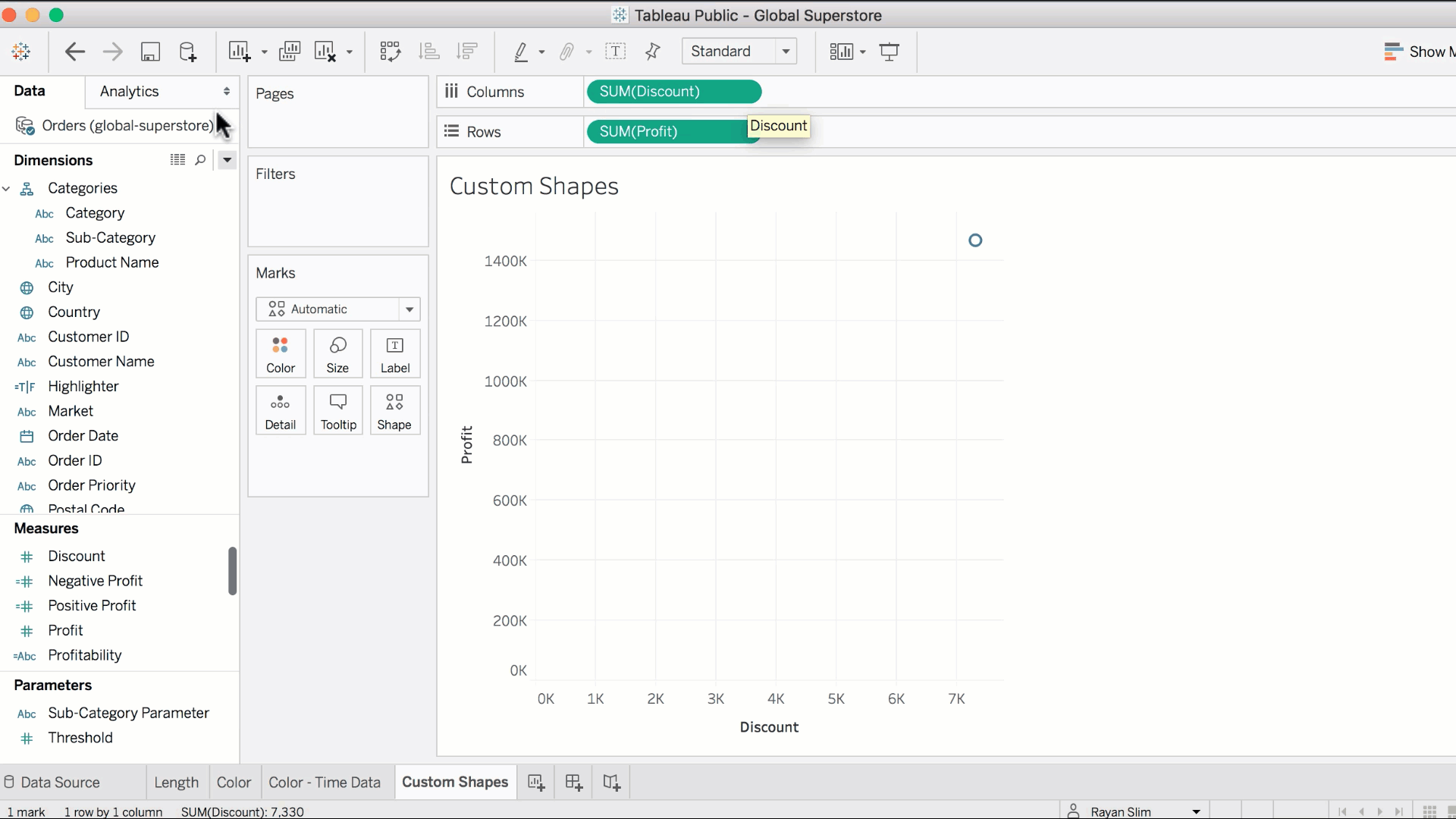Instead, we will take the average discount across all fields, and convert our decimals to percentages.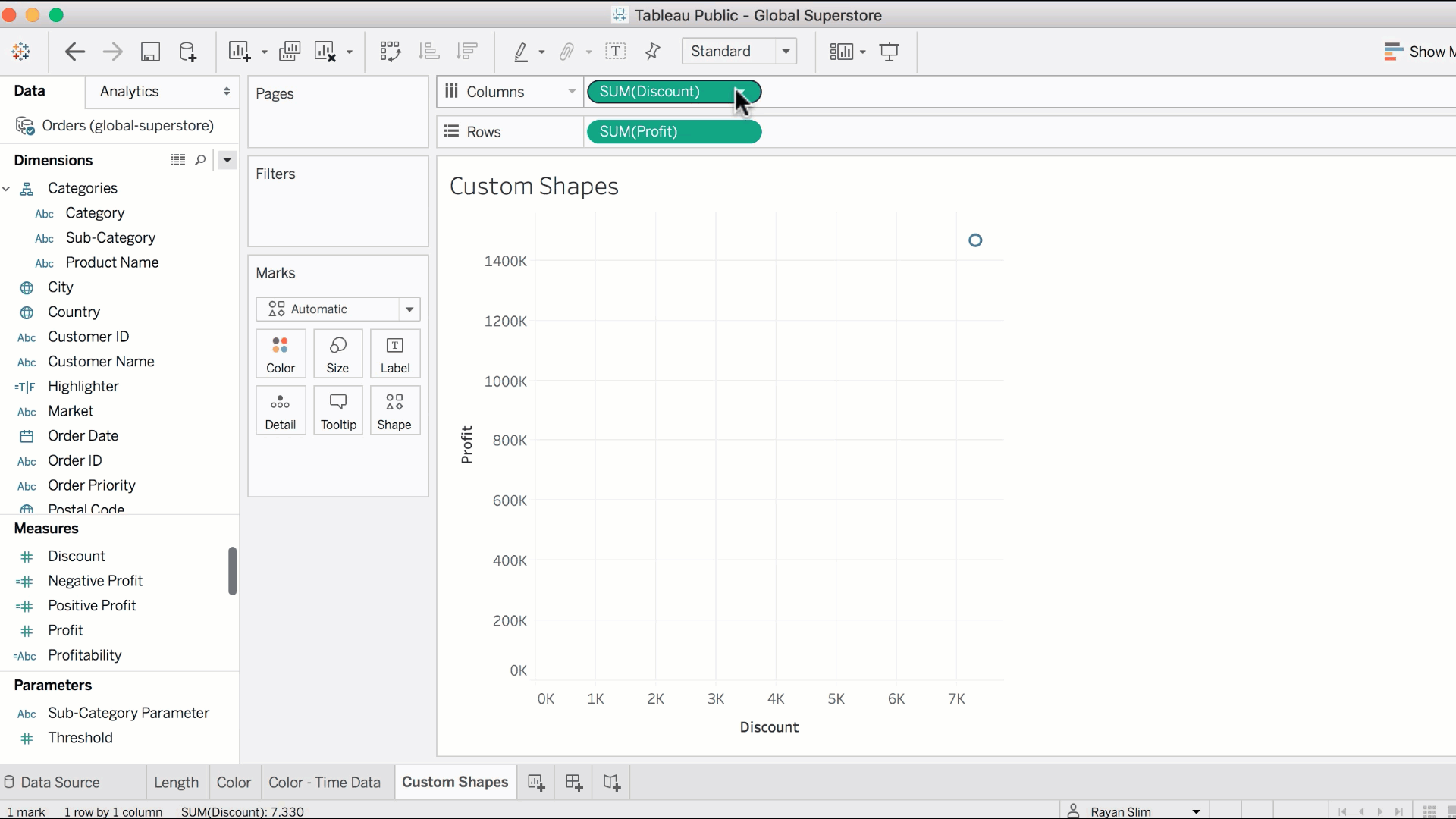This tells us that all the records were sold at an average discount of roughly 14%. Having only a single point on the scatter plot is not informative. To get more points, we simply need to increase the granularity. Rather than aggregating over all the records, we will aggregate the profits and discounts over each sub-category. This can be done by dragging sub-category to the detail mark.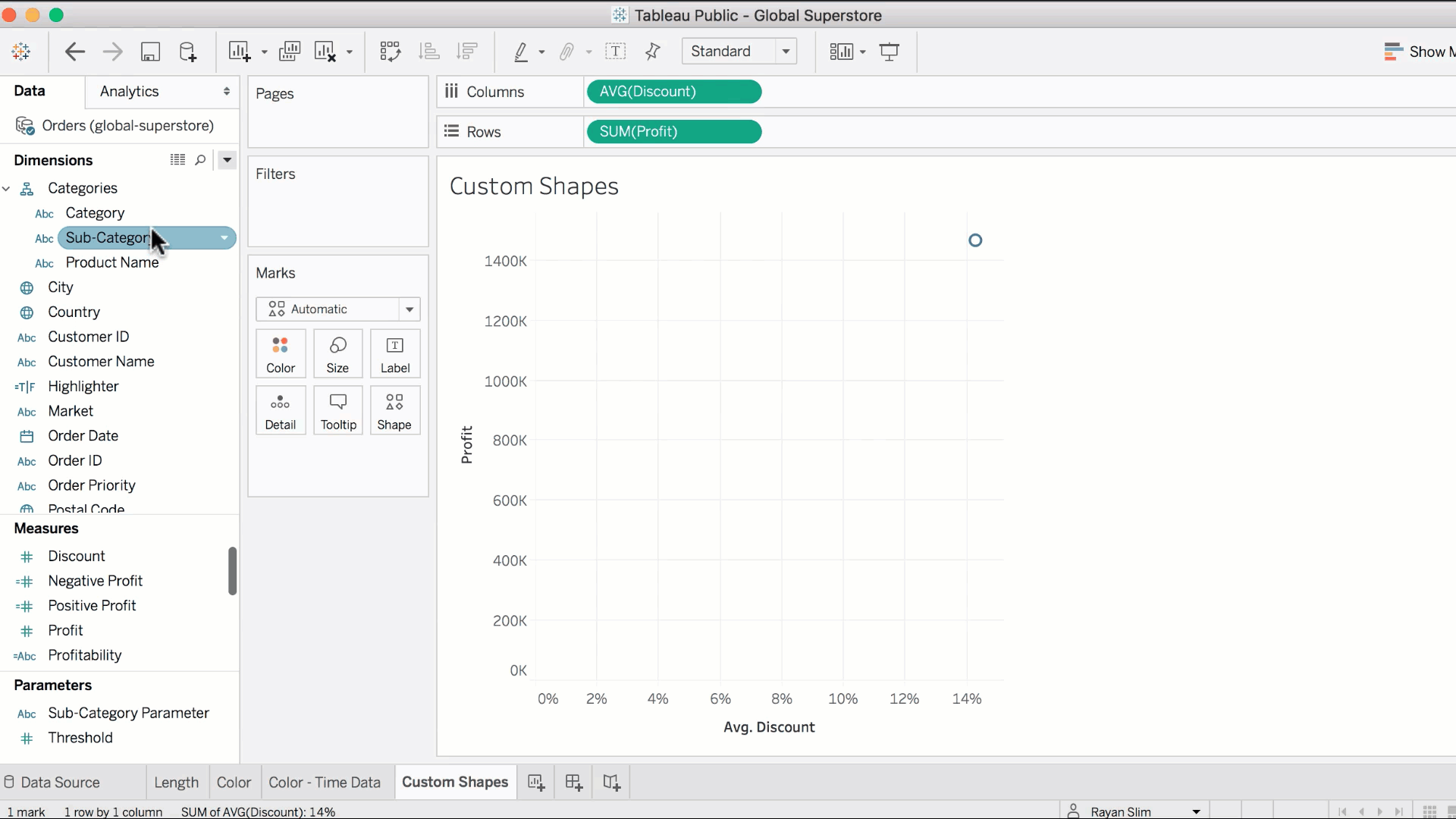Whenever you have two aggregated measures on the column and row shelves, you will need to use the detail mark to increase the level of detail at which the calculations are performed, as we just did.

## 2. Filters

It would make sense to analyze the relationship between profit and average discount one category at a time. Previously you filtered based on date, now we filter based on category. The process is the exact same. Simply drag the field that you're filtering by to the filter shelf.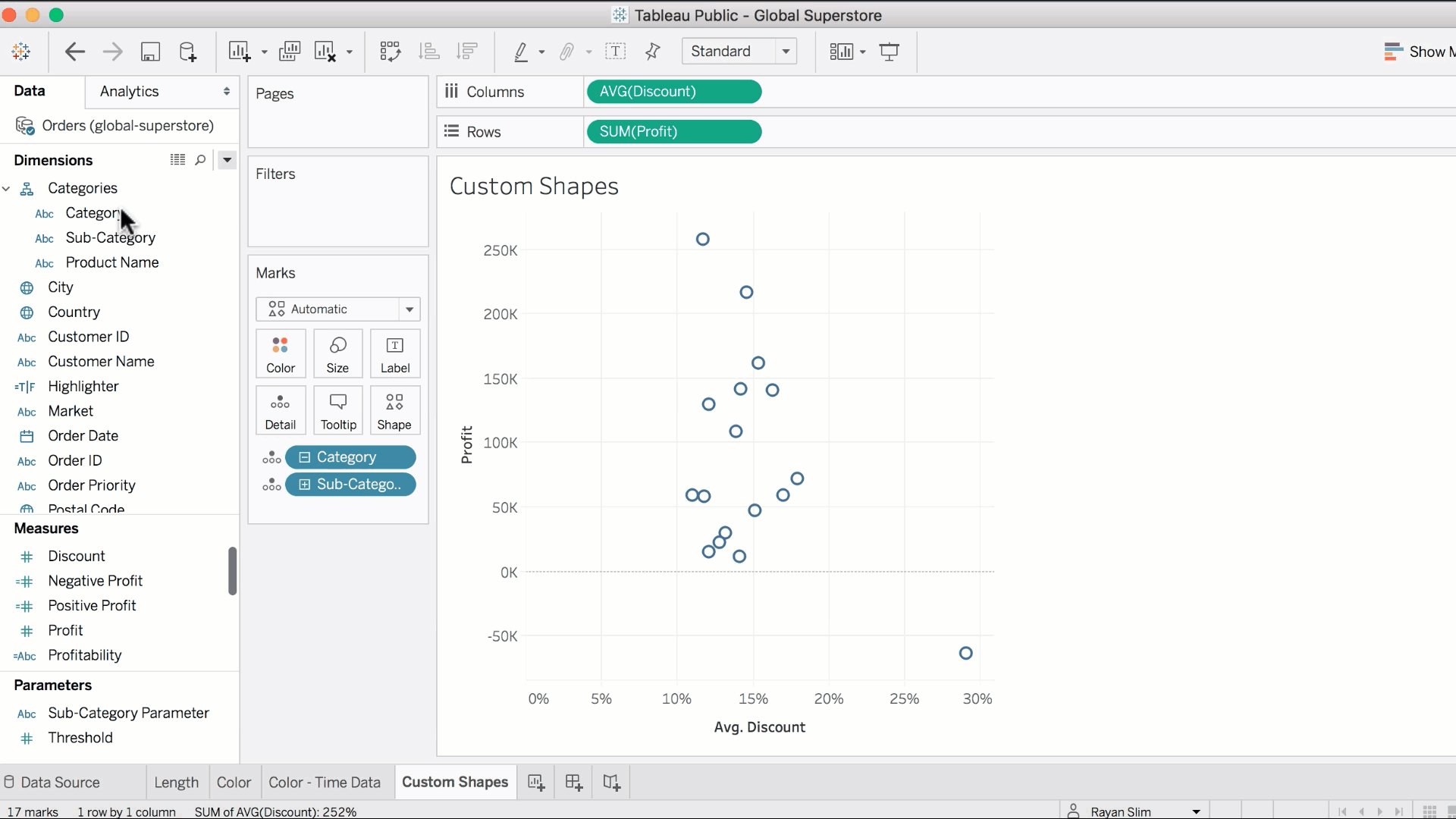Navigating through the filter, we can we can pick out that higher discount rates are a partial factor to losses in profit.Custom shapes are applied to this worksheet in lecture 25.

Feedback Summary
5.0
1 students
5

100%
4

0%
3

0%
2

0%
1

0%
Written Reviews
There are no written reviews yet.